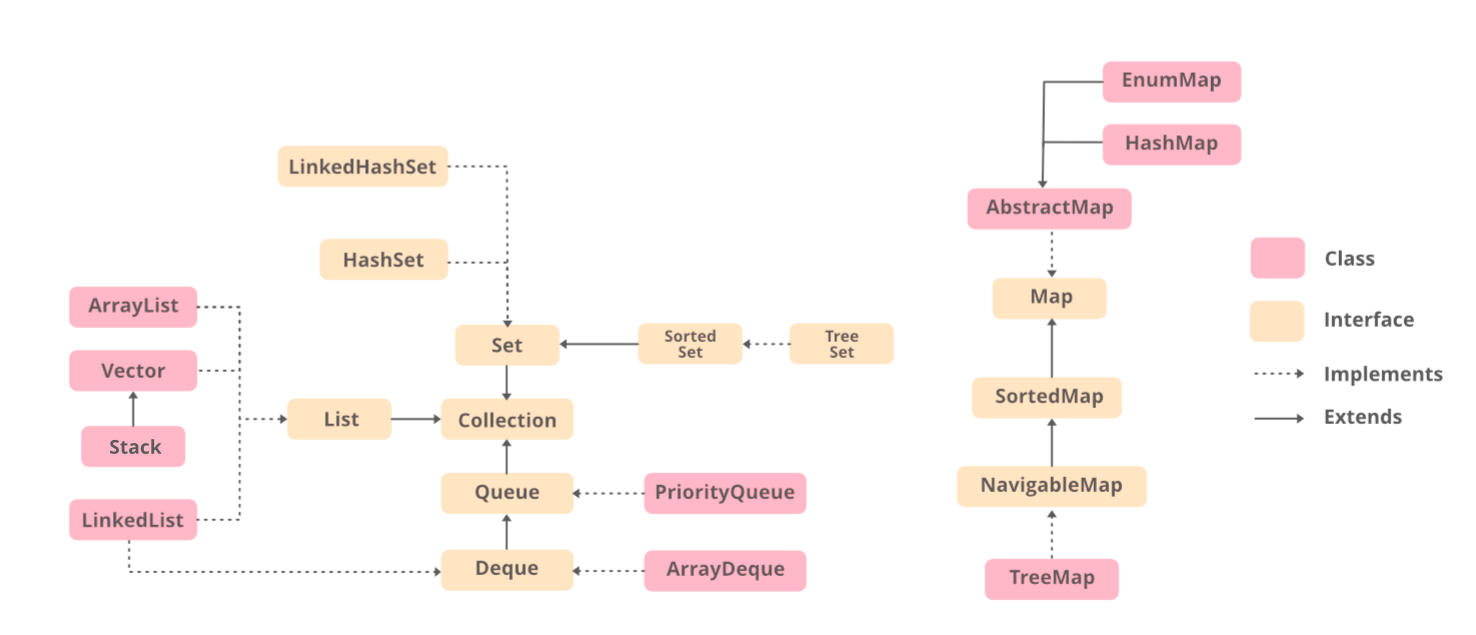# How to Loop Over TreeSet in Java?

• Difficulty Level : Easy
• Last Updated : 02 Nov, 2021

TreeSet is one of the most important implementations of the SortedSet interface in Java that uses a Tree for storage. The ordering of the elements is maintained by a set using their natural ordering whether or not an explicit comparator is provided. This must be consistent with equals if it is to correctly implement the Set interface

Now the task is to explore out how many ways are there in order to loop over TreeSet. As we all know TreeSet provides an implementation of the SortedSet Interface and SortedSet extends Set Interface. It behaves like a simple set with the exception that it stores elements in a sorted format.Following are some traits associated with TreeSet that are as follows:

• TreeSet uses tree data structure for storage.
• Objects are stored in sorted, ascending order. But we can iterate in descending order using the method TreeSet.descendingIterator().
• Access and retrieval times are very fast which make TreeSet an excellent choice for the storage of large volume of data in a sorted format.
• TreeSet doesn’t use hashCode() and equals() methods to compare it’s elements. It uses compare() (or compareTo()) method to determine the equality of two elements.

### Methods:

Below we  have listed various ways to iterate over the TreeSet in java which we are going to discuss further and will provide a clean java program for each of the following methods as follows:

1. Using Enhanced For loop
2. Using Iterator
3. Using streams (from Java8 onwards)

Method 1: Using Enhanced For loop

Enhanced For loop can be used to loop over the TreeSet in the below manner.

Syntax:

```for (Integer value : ts)
{
System.out.print(value);
}```

Example

## Java

 `// Java Program to Loop over TreeSet ` `// Using Enhanced For loop ` ` `  `// Importing required classes  ` `import` `java.util.Iterator; ` `import` `java.util.TreeSet; ` ` `  `// Main class ` `public` `class` `GFG { ` ` `  `    ``// Main driver method ` `    ``public` `static` `void` `main(String[] args) ` `    ``{ ` ` `  `        ``// Creating an empty TreeSet by ` `        ``// declaring object of TreeSet class of Integer type ` `        ``TreeSet ts = ``new` `TreeSet(); ` ` `  `        ``// Adding elements to above TreeSet object ` `        ``ts.add(``10``); ` `        ``ts.add(``61``); ` `        ``ts.add(``87``); ` `        ``ts.add(``39``); ` ` `  `        ``// Display message for better readability ` `        ``System.out.print(``"TreeSet: "``); ` ` `  `        ``// Looping over the TreeSet values ` `        ``for` `(Integer value : ts) ` ` `  `            ``// Print the values ` `            ``System.out.print(value + ``", "``); ` `       `  `        ``System.out.println(); ` `    ``} ` `}`

Output

`TreeSet: 10, 39, 61, 87, `

Method 2: Using Iterator

Iterator can be created over the TreeSet objects. Hence this iterator can be used to traverse or loop through the TreeSet.

Syntax:

```Iterator iterator = ts.iterator();

while (iterator.hasNext())
{
System.out.print(iterator.next());
}```

Example

## Java

 `// Java program to loop over TreeSet ` `// Using Iterator ` ` `  `// Importing required classes ` `import` `java.util.Iterator; ` `import` `java.util.TreeSet; ` ` `  `// Main class ` `public` `class` `GFG { ` ` `  `    ``// Main driver method ` `    ``public` `static` `void` `main(String[] args) ` `    ``{ ` ` `  `        ``// Creating an empty TreeSet by ` `        ``// declaring an object of TreeSet class ` `        ``TreeSet ts = ``new` `TreeSet(); ` ` `  `        ``// Adding elements to above object of TreeSet class ` `        ``// Using add() method ` `        ``ts.add(``10``); ` `        ``ts.add(``61``); ` `        ``ts.add(``87``); ` `        ``ts.add(``39``); ` ` `  `        ``// Create an Iterator over the TreeSet ` `        ``Iterator iterator = ts.iterator(); ` ` `  `        ``// Display message for better readability ` `        ``System.out.print(``"TreeSet: "``); ` ` `  `        ``// Looping over the TreeSet values ` `        ``while` `(iterator.hasNext()) ` ` `  `            ``// Print all the values inside TreeSet object ` `            ``System.out.print(iterator.next() + ``", "``); ` ` `  `        ``System.out.println(); ` `    ``} ` `}`

Method 3: Using Java 8 forEach / stream

Java 8 forEach / stream can be used to loop over the TreeSet in the below manner.

Syntax:

```Tree_Set.forEach(iterator -> System.out.print(i + " "));
// Using forEach ```
```Tree_Set.stream().map(iterator -> String.valueOf(i)).collect(Collectors.joining(", "))
// Using stream  ```

Example

## Java

 `// Java program to loop over TreeSet ` `// Using For-each and Stream in Java8 ` ` `  `// Importing required classes ` `import` `java.util.Arrays; ` `import` `java.util.Iterator; ` `import` `java.util.TreeSet; ` `import` `java.util.stream.Collectors; ` ` `  `// Main class ` `public` `class` `GFG { ` ` `  `    ``// Main driver method ` `    ``public` `static` `void` `main(String[] args) ` `    ``{ ` ` `  `        ``// Creating an empty TreeSet if integer type ` `        ``TreeSet ts = ``new` `TreeSet(); ` ` `  `        ``// Adding elements to the TreeSet ` `        ``// using addAll() method ` `        ``ts.addAll(Arrays.asList(``10``, ``61``, ``87``, ``39``)); ` ` `  `        ``// Looping over the TreeSet & print values ` `        ``System.out.print(``"TreeSet without Comma: "``); ` ` `  `        ``// Iterating through the TreeSet ` `        ``// using forEach ` `        ``ts.forEach(i -> System.out.print(i + ``" "``)); ` `        ``System.out.println(); ` ` `  `        ``// Looping over the TreeSet & print values ` `        ``System.out.print(``"TreeSet with Comma: "``); ` ` `  `        ``// Iterating through the TreeSet ` `        ``// Using stream concept introduced in Java8 ` `        ``System.out.print( ` `            ``ts.stream() ` `                ``.map(i -> String.valueOf(i)) ` `                ``.collect(Collectors.joining(``", "``))); ` `    ``} ` `}`

Output

```TreeSet without Comma: 10 39 61 87
TreeSet with Comma: 10, 39, 61, 87```

My Personal Notes arrow_drop_up
Recommended Articles
Page :